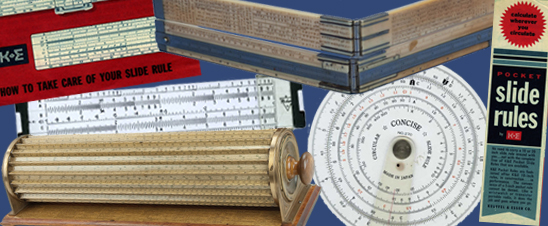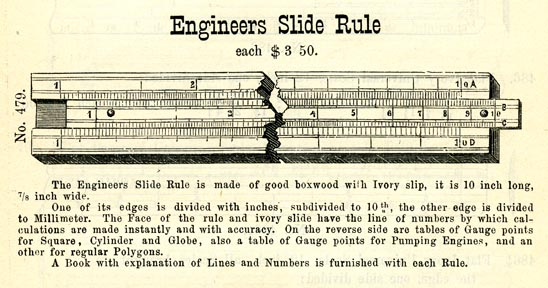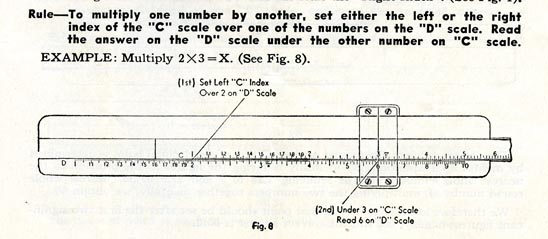# Slide Rules -- Linear Slide Rules

## Linear Slide RulesBetween 1614 and 1622, John Napier discovered logarithms, Edmund Gunter devised a scale on which numerals could be multiplied and divided by measuring the distance between two logarithmic numbers with a pair of dividers, and William Oughtred put two such scales alongside each other, moving one on a slide so that the distance between the numbers could be read off directly. The speed with which these developments unfolded suggests the power that logarithms provided for reducing the tedium of calculation. By the 1680s, the English used slide rules in carpentry and in gauging (estimating liquid volumes)—the instruments were quite helpful for determining excise taxes on barrels of liquor.

It was not until the late eighteenth century that slide rules were commonly utilized in the manufacture of machines and machine tools, most notably by James Boulton and James Watt. Several more decades passed before slide rule use became widespread throughout Europe. French artillery officer Amedée Mannheim fostered acceptance of the rectangular or linear form of the instrument in 1851 by standardizing the general types of scales and the order in which the scales were arranged. Mannheim also developed the cursor or indicator, which made it easier to read results from two scales that were not adjacent to each other. By the 1870s, German companies made slide rules one of the first consumer products to be fashioned out of plastic (specifically, sheets of celluloid laminated to a wooden frame), and they built dividing engines that permitted mass production of the scales engraved on slide rules. In the United States in the 1890s, Keuffel & Esser of New York City pioneered first the import and then the domestic manufacture of linear slide rules. Numerous other companies entered the market in the 20th century.Keuffel & Esser was one of the first American firms to import linear slide rules from Europe. Catalogue and Price List of Keuffel and Esser, 13th ed. (New York, 1880), 71. NMAH Trade Literature Collection, Smithsonian Institution Libraries. AHB2013q009220

A quick tour of what you can expect to see on a slide rule starts with the C and D scales, which both represent the standard number line. To multiply two numbers, set the 1 on the C scale above the first number to be multiplied on the D scale. Look at the second number to be multiplied on the C scale; the number below it on the D scale is the answer. For example, to multiply 2 by 3, set 1 on C over 2 on D, and then look below 3 on C to see the answer 6 on D. Division is accomplished by reversing the process. To calculate 6 ÷ 3, set 3 on C over 6 on D, then look at 1 on C to see the answer 2 on D. To deal with numbers larger than 10 or smaller than 1, the user must mentally move the decimal point. Slide rule users also had to be able to estimate distances between marks on the scales, since there was no way to include all of the digits needed to solve a problem such as 3.14 X 2.7. (On linear and circular slide rules, the answer is "approximately 8.48." Web sites on the Resources page provide more detailed training in using slide rules.)Instructions for multiplying two numbers on a slide rule. Eugene Dietzgen Co., Self-teaching Instruction Manual [for] Maniphase Slide Rule (Chicago, [1950s]), 4. NMAH Mathematics Collection, cat. no. 1988.0367.02. AHB2013q009216

The basic process for setting up and solving problems is the same for operations on other scales. If the numbers used in the calculation produce a result off the ends of the scales, a user employs the CI and DI scales, which put the number line in inverse, or reverse, order. If the numbers still extend past the end of the instrument, the user may try the "folded" CF and DF scales, which start numbering at π instead of at 1. The A and B scales are number lines of squares, so they are used with the C or D scales to square (or take the square root of) a number. The K scale provides cubes and cube roots. L scales represent common logarithms, S scales give sines and cosines, and T scales indicate tangents.

## Contents